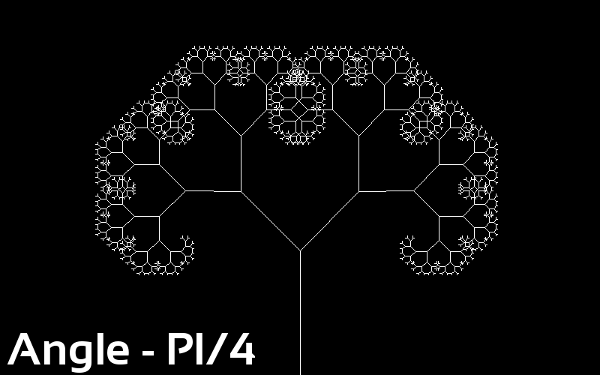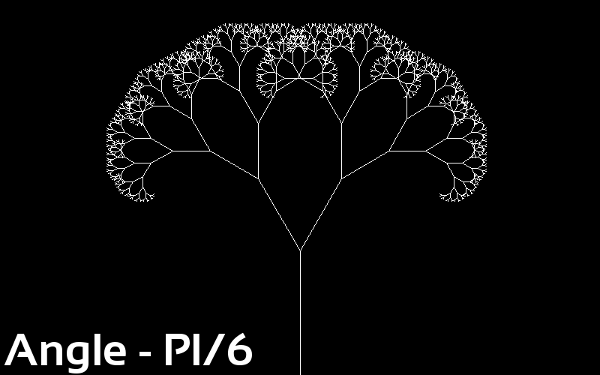### Fractals Basics : Recursion Trees (with Java Code)

Fractals & Recursion Trees

Fractals are self-similar patterns or detailed patterns repeating themselves. Fractals are one of the most beautiful because of their precise and repetitive nature. Examples of fractals are found abundantly in nature. Rivers, snow-flakes, frost to name few of them.Frost (Source: Wikipedia)Romanesco Brocolli (Source: Wikipedia)

One of the very basic figure that fulfills requirement of repetition is called a recursion tree.We draw a line, it divides into branches, then the branches further divide into still smaller ones until infinity. By now you may have guessed the reason of naming it a 'tree'. On a computer however we do not go on till infinity and stop the drawing when we reach satisfactory results.

In this post I illustrate the method of creating a Recursion tree with two branches. It can however be modified to add more branches with a small knowledge of Mathematics (Vectors).

As you may have guessed by now, we are going to use a recursive function (What is a recursive function? The one which calls itself.) to perform the task.

The Java Code

 ``` 1 2 3 4 5 6 7 8 9 10 11 12 13 14 15 16 17 18 19 20 21 22 23 24 25 26 27 28 29 30 31 32 33 34 35 36 37 38 39 40 41 42 43 44 45 46 47 48 49 50 51 52 53 54 55 56 57``` ```// @author Abdul Fatir // Email - abdulfatirs@gmail.com import java.io.*; import javax.imageio.*; import java.awt.*; import java.awt.image.*; import static java.lang.System.out; public class RecursionTree { private double ANGLE = Math.PI/10; private float SHRINK_FACTOR = 1.5f; private int MAX_RECURSIONS = 10; public void drawBranch(Graphics g,int pointX, int pointY,double directionX,double directionY, float size, int recursion_number) { // Finding the End Point of the Vector(line) to draw it int x2 = (int)(pointX + directionX*size), y2 = (int)(pointY + directionY*size); // Setting the Colour of the line g.setColor(Color.WHITE); // Drawing the line from initial to final point g.drawLine(pointX,pointY,x2,y2); // If the maximum recursions are reached we need to stop if(recursion_number>=MAX_RECURSIONS) return; // Finding the size of next branch by shrinking the current branch float new_branch_size = size/SHRINK_FACTOR; // Finding the Direction Vector of the next branch double directionX2 = Math.sin(ANGLE) * directionY + Math.cos(ANGLE) * directionX; double directionY2 = -(Math.sin(ANGLE) * directionX) + Math.cos(ANGLE) * directionY; // Incrementing the recursion_number by 1 int n2 = recursion_number+1; // Drawing the branch drawBranch(g,x2,y2,directionX2,directionY2,new_branch_size,n2); // Finding the Direction Vector of the corresponding branch on the other side i.e. with angle = -ANGLE directionX2 = Math.cos(-ANGLE) * directionX + Math.sin(-ANGLE) * directionY; directionY2 = -Math.sin(-ANGLE) * directionX + Math.cos(-ANGLE) * directionY; // Drawing the twin branch drawBranch(g,x2,y2,directionX2,directionY2,new_branch_size,n2); } public static void main(String args[])throws IOException { int IMG_WIDTH = 800; int IMG_HEIGHT = 500; // Creating a next 800x500 RGB Image BufferedImage img = new BufferedImage(IMG_WIDTH,IMG_HEIGHT,BufferedImage.TYPE_3BYTE_BGR); // Getting the graphics object to draw on Graphics g = img.getGraphics(); // Calling the drawBranch(Graphics, IntialX, InitialY, DirectionVectorX, DirectionVectorY, Length_Of_First_Line, Recursion_Number) new RecursionTree().drawBranch(g,IMG_WIDTH/2,IMG_HEIGHT,0,-1,IMG_HEIGHT/3,0); // Saving the Image ImageIO.write(img,"PNG",new File("tree.png")); } }  ```

The Java Code Explained

 ```11 12 13``` ```private double ANGLE = Math.PI/10; private float SHRINK_FACTOR = 1.5f; private int MAX_RECURSIONS = 10; ```

ANGLE is the angle (in radians) at which the new branches emerge with respect to the previous one.
SHRINK_FACTOR is the factor by which the size of next branch is reduced relative to the current one.
MAX_RECURSIONS is the maximum number of the times the function will call itself.

 `15` ```public void drawBranch(Graphics g,int pointX, int pointY,double directionX,double directionY, float size, int recursion_number) ```

The recursive function which draws a branch of the tree. It takes in the initial co-ordinate (pointX, pointY), the direction vector (directionX, directionY), the size/length of branch and the recursion number.

 ```17 18 19 20 21 22 23 24 25``` ```// Finding the End Point of the Vector(line) to draw it int x2 = (int)(pointX + directionX*size), y2 = (int)(pointY + directionY*size); // Setting the Colour of the line g.setColor(Color.WHITE); // Drawing the line from initial to final point g.drawLine(pointX,pointY,x2,y2); // If the maximum recursions are reached we need to stop if(recursion_number>=MAX_RECURSIONS) return; ```

Now we have the initial point, length and the direction vector of the branch. We find the final point of the line by multiplying the direction vector with length and adding it to the initial point. Then set the color WHITE and render the line on the image.

 ``` 26 27 28 29 30 31 32 33 34 35 36 37 38 39``` ```// Finding the size of next branch by shrinking the current branch float new_branch_size = size/SHRINK_FACTOR; // Finding the Direction Vector of the next branch double directionX2 = Math.sin(ANGLE) * directionY + Math.cos(ANGLE) * directionX; double directionY2 = -(Math.sin(ANGLE) * directionX) + Math.cos(ANGLE) * directionY; // Incrementing the recursion_number by 1 int n2 = recursion_number+1; // Drawing the branch drawBranch(g,x2,y2,directionX2,directionY2,new_branch_size,n2); // Finding the Direction Vector of the corresponding branch on the other side i.e. with angle = -ANGLE directionX2 = Math.cos(-ANGLE) * directionX + Math.sin(-ANGLE) * directionY; directionY2 = -Math.sin(-ANGLE) * directionX + Math.cos(-ANGLE) * directionY; // Drawing the twin branch drawBranch(g,x2,y2,directionX2,directionY2,new_branch_size,n2); ```

We find the length of next branch by dividing the length of current branch by the SHRINK_FACTOR. Find the direction vector of the next branch using basic trigonometry, increase the recursion number by 1 and call the function drawBranch again with the newly computed values.

Then in lines 36 and 37 we find the direction vector of the branch with angle on the opposite side (-ANGLE) and call drawBranch again.

The OutputsANGLE=Math.PI/2;ANGLE=Math.PI/4;ANGLE=Math.PI/6;ANGLE=Math.PI/10;
Beautifying the Output

We can randomize the color of every branch to get beautiful trees. Here is the modified drawBranch() function:

```public void drawBranch(Graphics g,int pointX, int pointY,double directionX,double directionY, float size, int recursion_number)
{
// Finding the End Point of the Vector(line) to draw it
int x2 = (int)(pointX + directionX*size), y2 = (int)(pointY + directionY*size);
// Setting the Colour of the line
java.util.Random random = new java.util.Random();
Color randomColor = new Color(random.nextInt(256), random.nextInt(256), random.nextInt(256));
g.setColor(randomColor);
// Drawing the line from initial to final point
g.drawLine(pointX,pointY,x2,y2);
// If the maximum recursions are reached we need to stop
if(recursion_number>=MAX_RECURSIONS)
{
return;
}
// Finding the size of next branch by shrinking the current branch
float new_branch_size = size/SHRINK_FACTOR;
// Finding the Direction Vector of the next branch
double directionX2  =  Math.sin(ANGLE) * directionY + Math.cos(ANGLE) * directionX;
double directionY2 = -(Math.sin(ANGLE) * directionX) +  Math.cos(ANGLE) * directionY;
// Incrementing the recursion_number by 1
int n2 = recursion_number+1;
// Drawing the branch
drawBranch(g,x2,y2,directionX2,directionY2,new_branch_size,n2);
// Finding the Direction Vector of the corresponding branch on the other side i.e. with angle = -ANGLE
directionX2  = Math.cos(-ANGLE) * directionX + Math.sin(-ANGLE) * directionY;
directionY2 = -Math.sin(-ANGLE) * directionX + Math.cos(-ANGLE) * directionY;
// Drawing the twin branch
drawBranch(g,x2,y2,directionX2,directionY2,new_branch_size,n2);

}
```

The output is: